# 不可不学的ROW函数（2）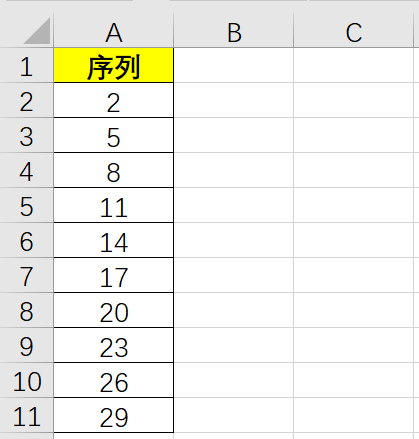A2单元格输入公式并下拉

=3*ROW(A1)-1

=d*ROW(A1)+(m-d)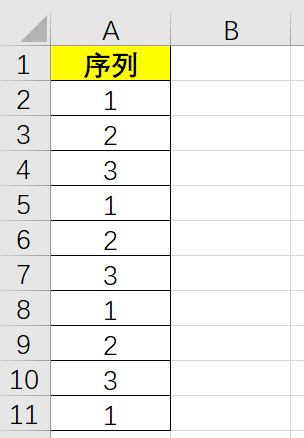A2单元格输入公式并下拉

=MOD(ROW(A1)-1,3)+1

=MOD(ROW(A1)-1,n)+m

=MOD(ROW(A1)-1,3)+1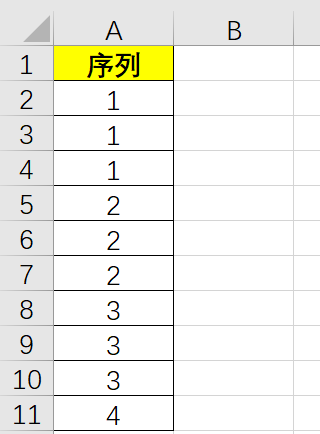A2单元格输入公式并下拉

=INT((ROW(A1)-1)/3)+1

=INT((ROW(A1)-1)/n)+m

=INT((ROW(A1)-1)/3)+1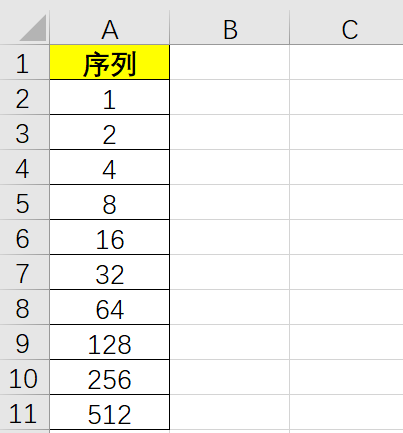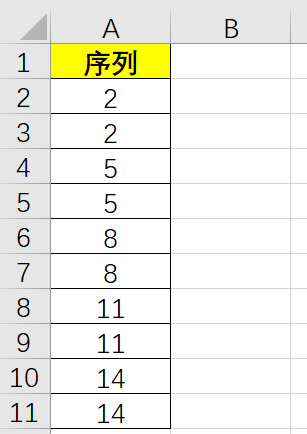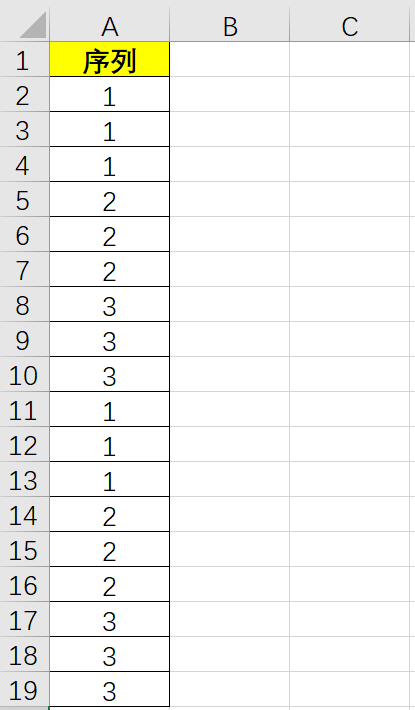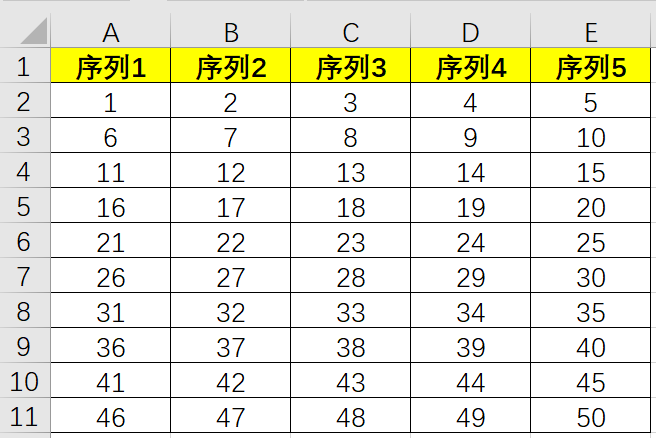1、首先独立思考必不可少，看见习题先独立思考，不懂就要问指的是独立思考之后还是不懂，不经过思考就提问那是对自己的不负责；

2、思考之后还不会怎么办？这个世界没有百度一下不会的，如果有，那就百度两下；

3、看了案例也不会做练习怎么办？那就扫描下方二维码关注“我的Excel”微信公众号和加入QQ群聊吧，公众号每天除了推送当天的练习之外也会推送前一天的练习答案，QQ群聊可以让众多Excel高手与你一对一交流。www.1cunzhang.com